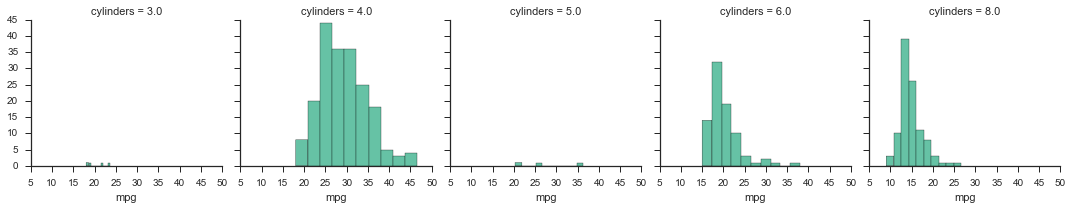# INTRODUCTION TO PYTHON FOR DATA MINING¶

Python is a great language for data mining. It has a lot of great libraries for exploring, modeling, and visualizing data. To get started I would recommend downloading the Anaconda Package. It comes with most of the libraries you will need and provides and IDE and package manager.

I do most of my work from the command line, but Anaconda comes with a launcher app that can be found in the ~/anaconda directory. To get the launcher to work with a Mac, you need to do the following:

1. Go to your terminal (hit command-space_bar and then type terminal)
2. Type conda install -f launcher
3. After that runs, type conda install -f node-webkit

Now you can open the launcher and see:

1. glueviz - This lets you link multiple plots across files
2. Ipython Notebook - A great way to display and work on your data mining projects
3. Ipython qtconsole - Basically an Ipython terminal for coding
4. Spyder - An IDE for Ipython

# IPython vs Python¶

Ipython is what makes Python interactive. Meaning that you can type some code, get some results, and then type some more code. This is very useful for exploring data because you don't always know what you are looking for and it can be annoying to have to run your entire program every time you make changes.

# Libraries You Should Know About¶

1. Pandas - Provides R like data structures and a high level API to work with data
2. Numpy - Provides fast numerical computing such as arrays and linear algebra
3. Scipy - For scientific computing such as drawing from distributions
4. Matplotlib - For plotting
1. Seaborn - To make your plots look better
5. Scikit-Learn - For machine learning; great documentation and tutorials
6. Statsmodels - For more traditional statistics

### Getting Seaborn¶

In the terminal type pip install seaborn

# An Example¶

I will use pandas to read in some data from the web and quickly remove the NA rows.

In :
import matplotlib.pyplot as plt
import numpy as np
from sklearn import datasets, linear_model
import pandas as pd
from pandas import DataFrame, Series
from __future__ import division
import seaborn as sns
from sklearn.cross_validation import train_test_split
sns.set(style='ticks', palette='Set2')
%matplotlib inline

names = ['mpg', 'cylinders', 'displacement', 'horsepower', 'weight', 'acceleration',
'model', 'origin', 'car_name'])
print(data.shape)
data = data.dropna()

(406, 9)

Out:
mpg cylinders displacement horsepower weight acceleration model origin car_name
0 18 8 307 130 3504 12.0 70 1 chevrolet chevelle malibu
1 15 8 350 165 3693 11.5 70 1 buick skylark 320
2 18 8 318 150 3436 11.0 70 1 plymouth satellite
3 16 8 304 150 3433 12.0 70 1 amc rebel sst
4 17 8 302 140 3449 10.5 70 1 ford torino

### Scikit-Learn¶

Here is a quick intro to modeling with scikit-learn. Basically you split the data into test and training. Then choose a model, fit the train data, and predict of the test data. Scikit-learn has great documentation, so check out their page.

In :
indep_vars = ['cylinders', 'displacement', 'horsepower', 'weight', 'acceleration']
dep_vars = ['mpg']
indep_data = data[indep_vars]
dep_data = data[dep_vars]
indep_train, indep_test, dep_train, dep_test = train_test_split(indep_data, dep_data, test_size=0.33, random_state=42)

In :
regr = linear_model.LinearRegression()
regr.fit(indep_train, dep_train)
print('Coefficients: {0}'.format(zip(indep_vars,np.squeeze(regr.coef_))))

Coefficients: [('cylinders', -0.26226222120889731), ('displacement', -0.0019562996653683384), ('horsepower', -0.059849640079272119), ('weight', -0.0049401742792735473), ('acceleration', 0.0040334885752014889)]

In :
regr_predict = regr.predict(indep_test)
print("Residual sum of squares: %.2f"
% np.mean((regr_predict - dep_test) ** 2))

Residual sum of squares: 19.71


### Tables¶

Some examples of how to use pandas to create summary statistics and tables

In :
data.groupby(['cylinders']).mpg.describe()

Out:
cylinders
3          count      4.000000
mean      20.550000
std        2.564501
min       18.000000
25%       18.750000
50%       20.250000
75%       22.050000
max       23.700000
4          count    199.000000
mean      29.283920
std        5.670546
min       18.000000
25%       25.000000
50%       28.400000
75%       32.950000
max       46.600000
5          count      3.000000
mean      27.366667
std        8.228204
min       20.300000
25%       22.850000
50%       25.400000
75%       30.900000
max       36.400000
6          count     83.000000
mean      19.973494
std        3.828809
min       15.000000
25%       18.000000
50%       19.000000
75%       21.000000
max       38.000000
8          count    103.000000
mean      14.963107
std        2.836284
min        9.000000
25%       13.000000
50%       14.000000
75%       16.000000
max       26.600000
dtype: float64
In :
pivot_table = data.pivot_table(index='cylinders', columns='acceleration', values='mpg', aggfunc=np.mean)

Out:
acceleration 8.0 8.5 9.0 9.5 10.0 10.5 11.0 11.1 11.2 11.3 ... 21.5 21.7 21.8 21.9 22.1 22.2 23.5 23.7 24.6 24.8
cylinders
3 NaN NaN NaN NaN NaN NaN NaN NaN NaN NaN ... NaN NaN NaN NaN NaN NaN NaN NaN NaN NaN
4 NaN NaN NaN NaN NaN NaN NaN NaN NaN NaN ... 43.1 44.3 30 19 24.5 29.0 23 43.4 44 27.2
5 NaN NaN NaN NaN NaN NaN NaN NaN NaN NaN ... NaN NaN NaN NaN NaN NaN NaN NaN NaN NaN
6 NaN NaN NaN NaN NaN NaN NaN NaN NaN 28.8 ... NaN NaN NaN NaN NaN NaN NaN NaN NaN NaN
8 14 14.5 14 15.5 14.5 17 13.285714 16 18.1 NaN ... NaN NaN NaN NaN NaN 23.9 NaN NaN NaN NaN

5 rows × 95 columns

### Plotting¶

Some examples creating some useful plots using matplotlib and seaborn. The despine function removes chart junk.

In :
p = plt.hist(data.mpg)
plt.title("MPG")
p
sns.despine()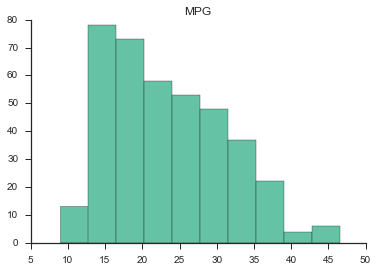In :
sns.lmplot("mpg", "weight", data);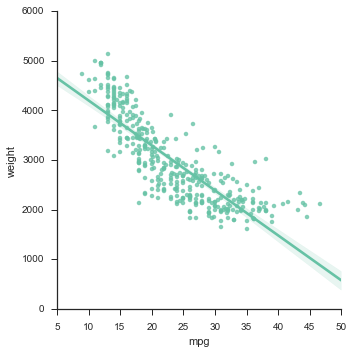In :
sns.lmplot("mpg", "weight", data, order=2);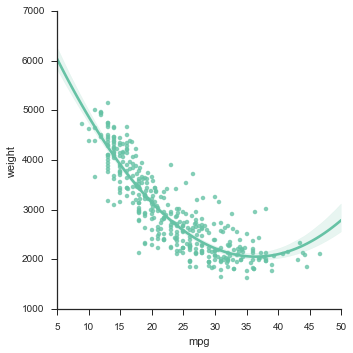In :
sns.jointplot("mpg", "weight", data, kind="reg")

Out:
<seaborn.axisgrid.JointGrid at 0x10f72c610>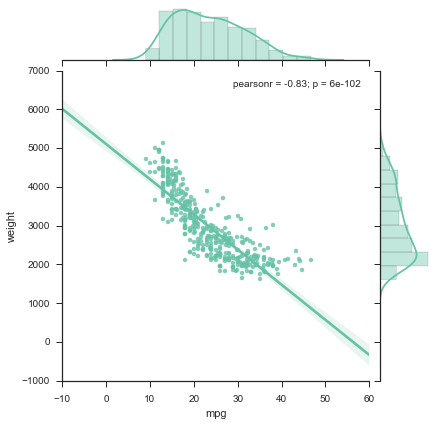In :
sns.boxplot(data[['displacement', 'horsepower']])

Out:
<matplotlib.axes._subplots.AxesSubplot at 0x10fc9a4d0>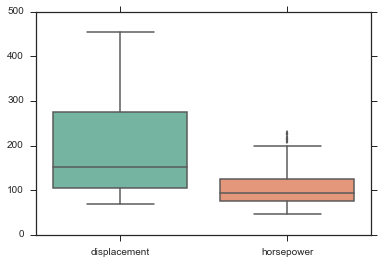In :
sns.violinplot(data[['displacement', 'horsepower']])

Out:
<matplotlib.axes._subplots.AxesSubplot at 0x10fdebfd0>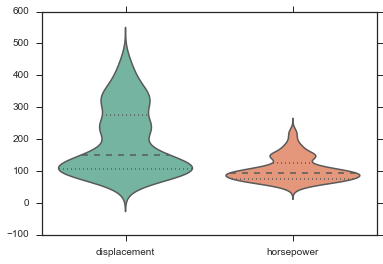In :
g = sns.FacetGrid(data, col="cylinders")
g.map(plt.hist, "mpg");